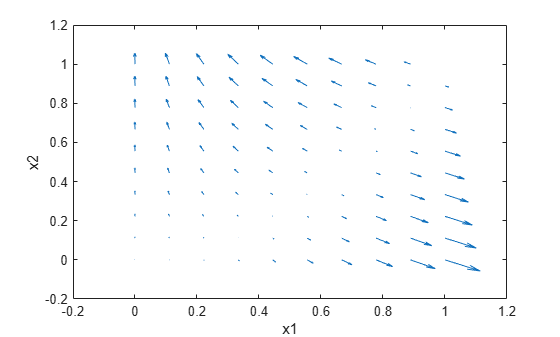Documentation

# dlfeval

Evaluate deep learning model for custom training loops

## Syntax

``[y1,...,yk] = dlfeval(fun,x1,...,xn)``

## Description

Use `dlfeval` to evaluate custom deep learning models for custom training loops.

### Tip

For most deep learning tasks, you can use a pretrained network and adapt it to your own data. For an example showing how to use transfer learning to retrain a convolutional neural network to classify a new set of images, see Train Deep Learning Network to Classify New Images. Alternatively, you can create and train networks from scratch using `layerGraph` objects with the `trainNetwork` and `trainingOptions` functions.

If the `trainingOptions` function does not provide the training options that you need for your task, then you can create a custom training loop using automatic differentiation. To learn more, see Define Custom Training Loops.

example

````[y1,...,yk] = dlfeval(fun,x1,...,xn)` evaluates the deep learning array function `fun` at the input arguments `x`1,…,`x`n. Functions passed to `dlfeval` can contain calls to `dlgradient`, which compute gradients from the inputs `x` by using automatic differentiation.```

## Examples

collapse all

Rosenbrock's function is a standard test function for optimization. The `rosenbrock.m` helper function computes the function value and uses automatic differentiation to compute its gradient.

`type rosenbrock.m`
```function [y,dydx] = rosenbrock(x) y = 100*(x(2) - x(1).^2).^2 + (1 - x(1)).^2; dydx = dlgradient(y,x); end ```

To evaluate Rosenbrock's function and its gradient at the point `[–1,2]`, create a `dlarray` of the point and then call `dlfeval` on the function handle `@rosenbrock`.

```x0 = dlarray([-1,2]); [fval,gradval] = dlfeval(@rosenbrock,x0)```
```fval = 1×1 dlarray 104 ```
```gradval = 1×2 dlarray 396 200 ```

Alternatively, define Rosenbrock's function as a function of two inputs, `x1` and x`2`.

`type rosenbrock2.m`
```function [y,dydx1,dydx2] = rosenbrock2(x1,x2) y = 100*(x2 - x1.^2).^2 + (1 - x1).^2; [dydx1,dydx2] = dlgradient(y,x1,x2); end ```

Call `dlfeval` to evaluate `rosenbrock2` on two `dlarray` arguments representing the inputs `–1` and `2`.

```x1 = dlarray(-1); x2 = dlarray(2); [fval,dydx1,dydx2] = dlfeval(@rosenbrock2,x1,x2)```
```fval = 1×1 dlarray 104 ```
```dydx1 = 1×1 dlarray 396 ```
```dydx2 = 1×1 dlarray 200 ```

Plot the gradient of Rosenbrock's function for several points in the unit square. First, initialize the arrays representing the evaluation points and the output of the function.

```[X1 X2] = meshgrid(linspace(0,1,10)); X1 = dlarray(X1(:)); X2 = dlarray(X2(:)); Y = dlarray(zeros(size(X1))); DYDX1 = Y; DYDX2 = Y;```

Evaluate the function in a loop. Plot the result using `quiver`.

```for i = 1:length(X1) [Y(i),DYDX1(i),DYDX2(i)] = dlfeval(@rosenbrock2,X1(i),X2(i)); end quiver(extractdata(X1),extractdata(X2),extractdata(DYDX1),extractdata(DYDX2)) xlabel('x1') ylabel('x2')```## Input Arguments

collapse all

Function to evaluate, specified as a function handle. If `fun` includes a `dlgradient` call, then `dlfeval` evaluates the gradient by using automatic differentiation. In this gradient evaluation, each argument of the `dlgradient` call must be a `dlarray` or a cell array, structure, or table containing a `dlarray`. The number of input arguments to `dlfeval` must be the same as the number of input arguments to `fun`.

Example: `@rosenbrock`

Data Types: `function_handle`

Function argument, specified as any MATLAB data type.

An input argument `x`j that is a variable of differentiation in a `dlgradient` call must be a traced `dlarray` or a cell array, structure, or table containing a traced `dlarray`. An extra variable such as a hyperparameter or constant data array does not have to be a `dlarray`.

Example: `dlarray([1 2;3 4])`

Data Types: `single` | `double` | `int8` | `int16` | `int32` | `int64` | `uint8` | `uint16` | `uint32` | `uint64` | `logical` | `char` | `string` | `struct` | `table` | `cell` | `function_handle` | `categorical` | `datetime` | `duration` | `calendarDuration` | `fi`

## Output Arguments

collapse all

Function output, returned as any data type. If the output results from a `dlgradient` call, the output is a `dlarray`.

## Tips

• A `dlgradient` call must be inside a function. To obtain a numeric value of a gradient, you must evaluate the function using `dlfeval`, and the argument to the function must be a `dlarray`. See Use Automatic Differentiation In Deep Learning Toolbox.

• `dlgradient` does not support higher order derivatives. In other words, you cannot pass the output of a `dlgradient` call into another `dlgradient` call.

• To enable the correct evaluation of gradients, the function `fun` must use only supported functions for `dlarray`. See List of Functions with dlarray Support.